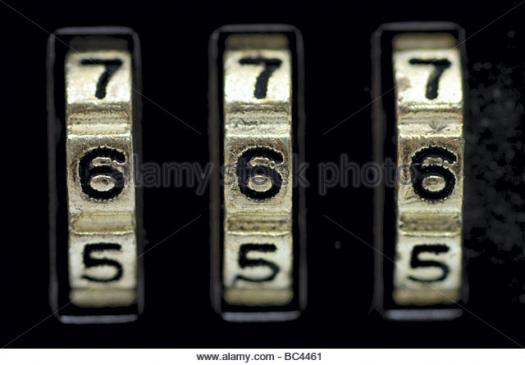# Do You Know Two-step Equations Intro?

Approved & Edited by ProProfs Editorial Team
At ProProfs Quizzes, our dedicated in-house team of experts takes pride in their work. With a sharp eye for detail, they meticulously review each quiz. This ensures that every quiz, taken by over 100 million users, meets our standards of accuracy, clarity, and engagement.
| Written by Livyn
L
Livyn
Community Contributor
Total Contribution - 348 | Total attempts - 140,973
Questions: 10 | Attempts: 132SettingsDo you know two-step equations intro? It is an equation or inequality that has more than two operations. Find out by taking this quiz.

• 1.

### It takes how many steps to solve an equation or inequality that has more than one operation?

• A.

Two

• B.

One

• C.

Three

• D.

Four

A. Two
Explanation
To solve an equation or inequality that has more than one operation, it typically takes two steps. The first step is to simplify the equation or inequality by applying the order of operations and combining like terms. This step involves performing any necessary addition, subtraction, multiplication, or division. The second step is to isolate the variable by undoing the operations in reverse order. This may involve performing addition or subtraction to move constant terms to the other side of the equation, or performing multiplication or division to cancel out coefficients. Therefore, it takes two steps to solve an equation or inequality with multiple operations.

Rate this question:

• 2.

### Two-step equations is a topic under ...

• A.

Decimals

• B.

Ratio

• C.

Equations

• D.

Variables

C. Equations
Explanation
Two-step equations is a topic under equations. In mathematics, equations are mathematical statements that contain an equal sign and express the equality of two expressions. Two-step equations are equations that require two operations to solve, usually involving addition, subtraction, multiplication, or division. This topic focuses on understanding and solving equations that involve multiple steps to find the value of the variable.

Rate this question:

• 3.

### Equations is a topic under ...

• A.

Music

• B.

Biology

• C.

Maths

• D.

Sociology

C. Maths
Explanation
Equations is a topic that falls under the subject of maths. In mathematics, equations are used to represent relationships between variables and to solve problems. Equations involve the use of symbols, numbers, and operations to express mathematical relationships and find unknown values. Therefore, the correct answer is maths.

Rate this question:

• 4.

### Mathematics is studied in the ...

• A.

Church

• B.

Mosque

• C.

School

• D.

Museum

C. School
Explanation
Mathematics is studied in schools because it is a fundamental subject that provides the foundation for various other fields of study. Schools provide a structured environment where students can learn and develop their mathematical skills. Additionally, schools have qualified teachers who can guide and support students in their mathematical journey. Mathematics is also an essential part of the curriculum in schools as it helps students develop critical thinking, problem-solving, and logical reasoning skills, which are important for their overall academic and professional growth.

Rate this question:

• 5.

### ...... is not a scientist

• A.

Albert Einstein

• B.

Isaac Newton

• C.

Will Smith

• D.

Bill Nye

C. Will Smith
Explanation
Will Smith is not a scientist. While Albert Einstein and Isaac Newton are renowned physicists, and Bill Nye is a science educator and television host, Will Smith is primarily known as an actor and rapper.

Rate this question:

• 6.

### In two-step equation, simplify using the inverse of addition or .....

• A.

Subtraction

• B.

Division

• C.

Multiplication

• D.

None

A. Subtraction
Explanation
In a two-step equation, the goal is to isolate the variable by performing inverse operations. In this case, the correct answer is subtraction because by subtracting a number from both sides of the equation, we can eliminate it from one side and simplify the equation. This helps in isolating the variable and solving for its value.

Rate this question:

• 7.

### Then simplify further by using the inverse of multiplication or ....

• A.

Subtraction

• B.

Division

• C.

• D.

None

B. Division
Explanation
To simplify further by using the inverse of multiplication, we would need to divide. Division is the inverse operation of multiplication, where we divide one number by another to find the quotient. Therefore, the correct answer is division.

Rate this question:

• 8.

### One goal in solving an equation is to have only variables on .... side of the equal sign.

• A.

One

• B.

Two

• C.

Three

• D.

Four

A. One
Explanation
In solving an equation, the goal is to isolate the variable on one side of the equal sign. This allows us to determine the value of the variable by performing operations on the other side of the equation. Having only variables on one side simplifies the equation and makes it easier to solve.

Rate this question:

• 9.

### One goal in solving an equation is to have only variables on one side of the equal sign and numbers on the other side of the ....

• A.

Equal sign

• B.

Coefficient

• C.

Constant

• D.

Variable

A. Equal sign
Explanation
In solving an equation, the goal is to isolate the variable on one side of the equal sign and have the numbers on the other side. The equal sign acts as the balance point, indicating that the expressions on both sides are equal. Therefore, the correct answer is "equal sign."

Rate this question:

• 10.

### The other goal is to have the number in front of the .... equal to one.

• A.

Proportion

• B.

Variables

• C.

Constant

• D.

CoefficientBack to top